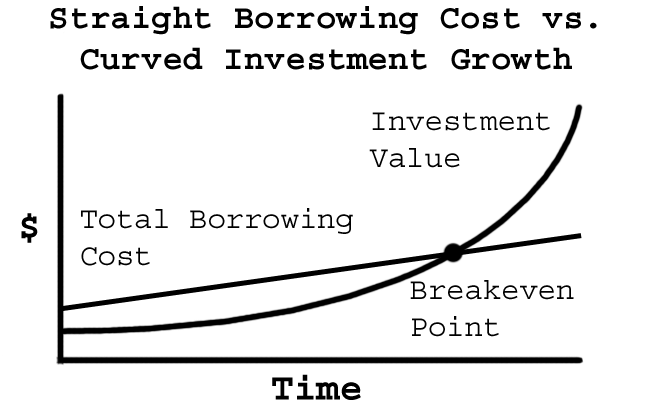﻿ Talbot Stevens

STRATEGY SHEET

July 1996Leverage Breakeven Point
Lower Than You Think

Leverage — borrowing to invest — is controversial because, like a power tool, leverage can produce both tremendous gains or damage, depending on how it is used.

Understanding the real breakeven point of leverage is critical because mathematically, the breakeven point of any investment strategy defines the risk of that strategy.

Most people reasonably think that their investment returns must exceed their cost of borrowing for leverage to be profitable. This is not true for several reasons.

Tax Benefits. Generally, when you borrow to invest, the interest expense is tax deductible, which lowers your real cost of borrowing and hence the breakeven point.

Capital gain investments, like equity mutual funds, have additional tax benefits because they compound tax-deferred until sold for a profit, and then you only pay tax on three-quarters of your gains.

Straight line vs. curved line. Let's assume that you pay 10% interest to borrow \$10,000. Your interest expense for year one is \$1,000, the same as for year two, year nine, etc. Thus the total interest cost increases by the same amount each year.

Technically, debt interest charges are called simple interest, because they are the same every year. When graphed, the total borrowing cost produces a straight line.

However, investments compound, with interest on interest. This compound interest when graphed, produces a curved line.

This subtle distinction — that debt is simple interest with a straight line cost while investments compound with a curved line — has huge implications.Even ignoring the tax benefits, you do not need to even clear your cost of borrowing to breakeven because an investment that grows at a lower rate than your cost of borrowing will eventually cross the cost line.

Also, the breakeven point for leverage decreases over time. Thus, by having a long-term time frame, the risk that leverage won't be profitable decreases, while the probability of achieving the desired equity returns increases. Both of these factors should help the investor hang on through the natural short-term fluctuations of equities.

Next month's Strategy Sheet will quantify how low the real breakeven point is, and how it — and hence your risk with leveraging — decreases over time.